# Quick Sort in C++: The Complete Guide

Like Merge Sort, Quick Sort is also a recursive sorting algorithm that uses Divide and Conquers method. Please read our Merge Sort tutorial first if you don’t know what Divide and Conquer are.

British computer scientist Tony Hoare developed the QuickSort algorithm in 1959 and published it in 1961. Quicksort is the algorithm used in most of the compilers in their sort().

## Quick Sort in C++

In C++ Quick Sort, first, we need to choose a pivot value (preferably the last element of the array). Then, we arrange the smaller values towards the left side of the pivot and higher values towards the right side of the pivot.

These two operations are performed recursively until only one element is left at both sides of the pivot. In other words, the quicksort algorithm is the following.

QuickSort is a sorting algorithm that is commonly used in computer science. For example, QuickSort is a divide and conquers algorithm.

It creates two empty arrays to hold elements less than the pivot value and elements more significant than the pivot value and then recursively sort the sub-arrays.

There are two basic operations in the algorithm, swapping items in place and partitioning the section of an array.

## Example of QuickSort Algorithm

Now, see the following example.

Suppose we are given an array.

 15 85 32 4 21 24

So here, our pivot =24. Now, the array will follow after arranging all smaller elements to the left side of 24 and more significant elements to the right side of 24.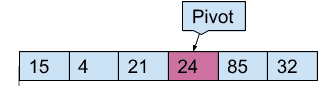So after the first pass, 24 is placed in its correct position. Now, we will again perform the partition operation to the left sub-array and the right sub-array, and so on.

So, the operations will be at each step like the following.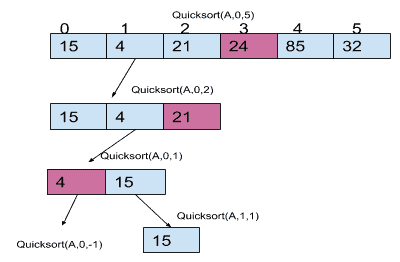What happened here is:

First, it was called Quicksort(Array,start_index,end_index), then the partition() was called, and it arranged the smaller number at the left side of the pivot and higher numbers at the right side of the pivot. And again, Quicksort() was called recursively. So in this way, we got 15 at last.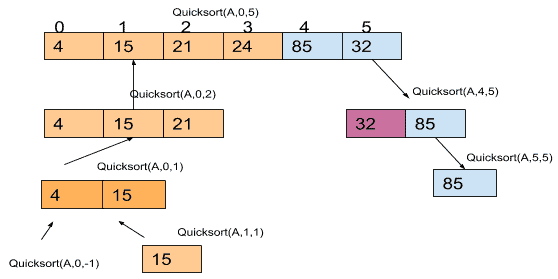After that, the Quicksort() returned the sorted subarray at each step, and then again, the operations on the right subarray were performed like the below image.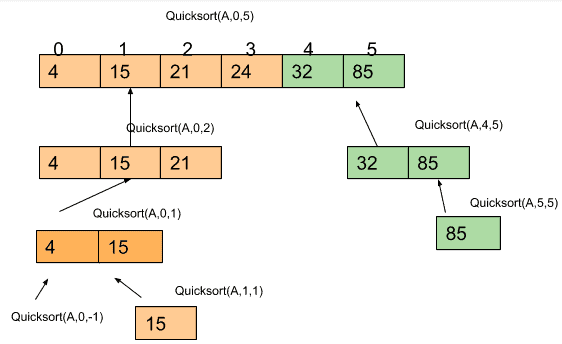So, here I have shown you how Quicksort is performed recursively in these three pictures.

1. Find a “pivot” element in an array. The pivot item is the basis for comparison for a single round.
2. Start the pointer (the left pointer) at the first item in the array.
3. Start the pointer (the right pointer) at the last item in the array.
4. While the value at the left pointer in the array is less than the pivot value, move the left pointer to the right (add 1). Continue until the value at the left pointer is greater than or equal to the pivot value.
5. While the value at the right pointer in the array is greater than the pivot value, move the right pointer to the left (subtract 1). Continue until the value at the right pointer is less than or equal to the pivot value.
6. If the left pointer is less than or equal to the right pointer, swap the values in an array at these locations.
7. Move the left pointer to the right by one and the right pointer to the left by one.
8. If the left pointer and right pointer don’t meet, go to step 1.

## Pseudo Code for Quicksort Algorithm

QUICKSORT(A, start, end)

1. if start < end
2. P_indx =PARTITION(A, start, end)
3. QUICKSORT(A, start, P_indx-1)
4. QUICKSORT(A, P_indx +1, end)
5. Exit

PARTITION(A, start, end)

1.  Set pivot = A[end]
2. Set P_indx = start
3. Repeat steps 4 to 7 for j = start to end – 1
4. if A[j]<=pivot
5. exchange A[P_index] with A[j]
6. Set P_index=P_index+1

[End of If block]

1. exchange A[P_index] with A[end]

[End of For Loop]

8. return P_index

## C++ QuickSort Program

See the below C++ Quicksort code.

```#include<bits/stdc++.h>
using namespace std;
int partition(int *a,int start,int end)
{
int pivot=a[end];
//P-index indicates the pivot value index

int P_index=start;
int i,t; //t is temporary variable

//Here we will check if array value is
//less than pivot
//then we will place it at left side
//by swapping

for(i=start;i<end;i++)
{
if(a[i]<=pivot)
{
t=a[i];
a[i]=a[P_index];
a[P_index]=t;
P_index++;
}
}
//Now exchanging value of
//pivot and P-index
t=a[end];
a[end]=a[P_index];
a[P_index]=t;

//at last returning the pivot value index
return P_index;
}
void Quicksort(int *a,int start,int end)
{
if(start<end)
{
int P_index=partition(a,start,end);
Quicksort(a,start,P_index-1);
Quicksort(a,P_index+1,end);
}
}
int main()
{
int n;
cout<<"Enter number of elements: ";
cin>>n;
int a[n];
cout<<"Enter the array elements:\n";
for(int i=0;i<n;i++)
{
cin>>a[i];
}
Quicksort(a,0,n-1);
cout<<"After Quick Sort the array is:\n";
for(int i=0;i<n;i++)
{
cout<<a[i]<<" ";
}
return 0;
}
```

The output is below.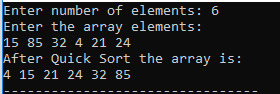## Time Complexity for Quicksort

The worst case occurs when the partition process always picks the greatest or smallest item as a pivot and the complexity of Worst Case: O(N^2).

The best-case occurs when a partition process always picks the middle element as a pivot. And the complexity of Best Case: O(N).

The average case means when there is neither a balanced tree nor the Worst Case. The complexity of the Average Case: (N log N).

## Why Quicksort over Merge Sort?

Time Complexity for QuickSort is O(n2). Its average-case running time is O(nlog(n)), but its worst-case is O(n2), which occurs when you run it on the list that contains a few unique items.

Randomization takes O(n). But, of course, it doesn’t change its worst case, and it just prevents the malicious user from making your sort take a long time.

QuickSort is more popular because it:

1. It is in place (Merge Sort requires extra memory linear to several elements to be sorted).
2. It has a small hidden constant.

That’s it for the C++ Quick Sort.

Categories C++

### 1 thought on “Quick Sort in C++: The Complete Guide”

1.Thanks a lot